# The sum 13

The sum of the exponents of the prime factors in the prime factorization of 196 is. .. .

s =  4

### Step-by-step explanation: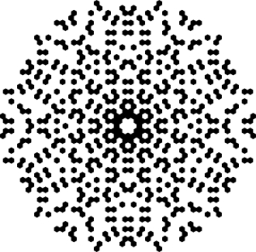Did you find an error or inaccuracy? Feel free to write us. Thank you!## Related math problems and questions:

• Prime factorsWrite 98 as a product of prime factors
• Statistics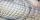The sum of all deviations from the arithmetic mean of the numerical sequence 4, 6, 51, 77, 90, 93, 95, 109, 113, 117 is:
• We bought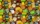We bought 140 fruit, 196 chocolate and 84 caramel lollipops. How many of the same packages can we prepare from them?
• Bundle of candies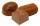In the store has 168 chocolates, caramel candies 224 and 196 hard candies. How many packages we can do and how many of candies will be in each package?
• RemaindersIt is given a set of numbers { 170; 244; 299; 333; 351; 391; 423; 644 }. Divide this numbers by number 66 and determine set of remainders. As result write sum of this remainders.
• The ticketsThe tickets to the show cost some integer number greater than 1. Also, the sum of the price of the children's and adult tickets, as well as their product, was the power of the prime number. Find all possible ticket prices.
• Comparing exponents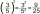A) 8 (6²)(8²) b) 16 (6²)(8²) c) (6¹+²) (8²)
• Sum of the seventeen numbersThe sum of the 17 different natural numbers is 154. Determine the sum of the two largest ones.
• Find the sumFind the sum of all natural numbers from 1 and 100, which are divisible by 2 or 5
• Divisors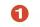Find all divisors of number 493. How many are them?
• Decomposition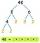Make decomposition using prime numbers of number 155. Result write as prime factors (all, even multiple)
• 7 digit numberIf 3c54d10 is divisible by 330, what is the sum of c and d?
• Prime divisorsFind 2/3 of the sum's ratio and the product of all prime divisors of the number 120.
• The sumThe sum of the squares of two immediately following natural numbers is 1201. Find these numbers.
• Sum of odd numbersFind the sum of all odd integers from 13 to 781.
• Sum and ratioThe sum and ratio of two numbers is equal to 10. Which numbers are they?
• Prime factorsFactor the number 6600 into the product of prime numbers.